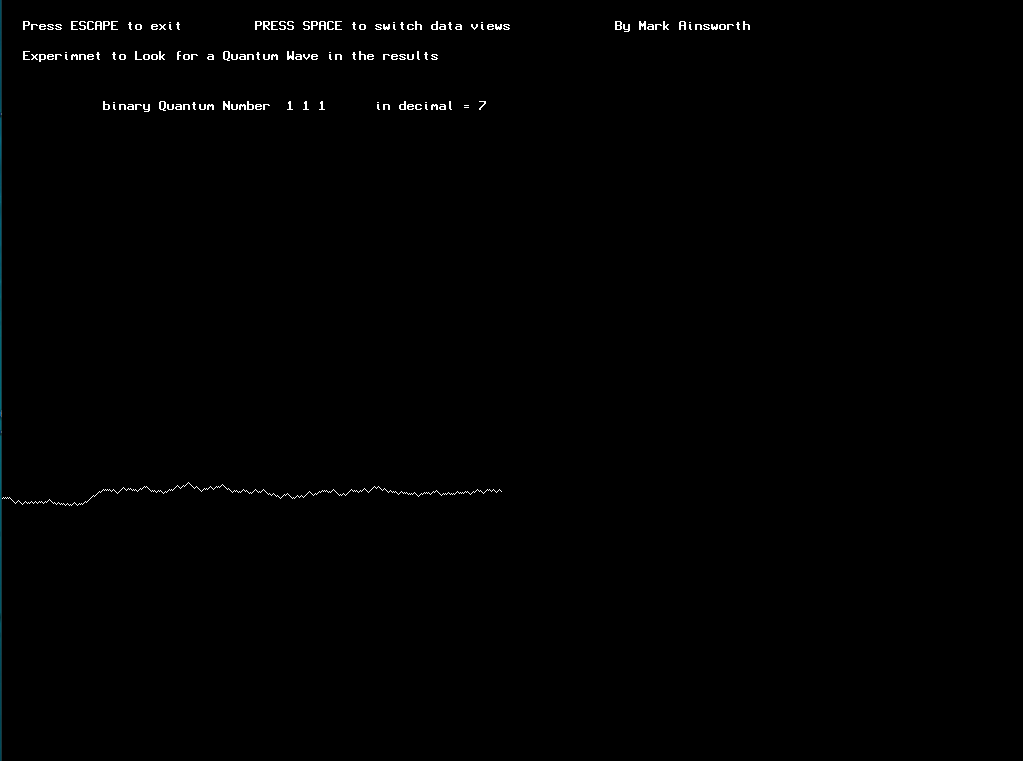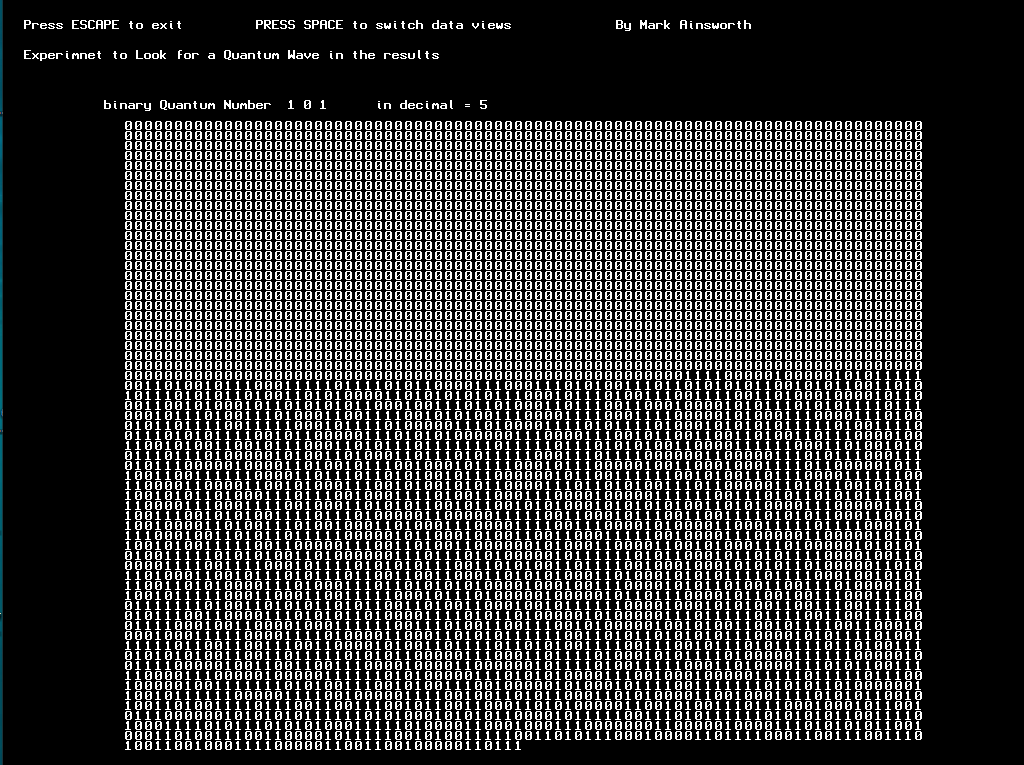January 21, 2021, 07:25:46 AM

###Author Topic: Quantum Mechanics Wave generator Experiment coded in blitzmax  (Read 1133 times)

####mainsworthy

• Jr. Member
•• Posts: 44##### Quantum Mechanics Wave generator Experiment coded in blitzmax
« on: October 24, 2019, 01:19:57 AM »https://globebotter.itch.io/quantum-mechanics-experiment-app

Graphics 1024,768
' "Quantum Ainsworth experiment in blitzmax version 01" for us simple folk to understand Quantum theory By Mark Ainsworth
'Quatum Math on off phased 1 bit registers with 3 binary bit numbers from 0 to 7
'
'Quantum Math uses numbers that can be on or off BUT you never know if they are on or off, I'm using 3 variables reg1 reg2 & reg3, I am randomly swithching on off
'
'I am flling an array called list[] with the result of the three quantum variables, then I am displaying the last 5000/3 results in sets of 3
'
'In this experiment Im looking for the Quantum wave in the data, it only displays 5000/3 numbers, so it would be hard to see, but this app should give you enough to create your own experimnent

Global dataview = 1

'these are the 3 quantum memory banks
Global reg1 = 0
Global reg2 = 0
Global reg3 = 0

'tot can be used when looking a decimal
Global tot = 0
' theses three are the random switches to power the quantum maths
Global qq = 0
Global qq2 = 0
Global qq3 = 0

'just display coordinates
Global x = 0
Global y = 0

'list[] stores the results
Global list
'count is just a count of how many results have been generated & tells the loop to display the last 5000 results
Global count = 5000

'while loop
While Not KeyHit(KEY_ESCAPE)

Cls
If KeyHit(KEY_SPACE)
dataview = dataview + 1
If dataview > 1 Then dataview = 0
EndIf
' tot add the quantum binary numbers together into decimal, its not used yet
tot = 0
tot = (reg3 *4) + (reg2 *2) + reg1

'display quantum memory, they are always in a state of on off
DrawText "Press ESCAPE to exit         PRESS SPACE to switch data views             By Mark Ainsworth",20,20
DrawText "Experimnet to Look for a Quantum Wave in the results",20,50
DrawText  "binary Quantum Number  "+reg3+" "+reg2+" "+reg1+"      in decimal = "+tot,100,100

'the switches to randomly turn the quantum registers on off
qq = Rand(1,2)
qq2 = Rand(1,2)
qq3 = Rand(1,2)
If qq = 1 Then q1()
If qq2 = 1 Then q2()
If qq3 = 1 Then q3()

' store results in list[]
If qq = 1 Or qq2 = 1 Or qq3 = 1
count = count + 3
'list[count] = tot
list[count] = reg1
list[count-1] = reg2
list[count-2] = reg3
EndIf

'loop to display the results
x = 0
y = 0
If dataview = 0
For f = count - 5000 To count - 1
DrawText ""+list[f],(x*10)+121,(y*10)+121
x = x + 1
If x = 80
y = y + 1
x = 0
EndIf
Next

EndIf

If dataview = 1
x = 0
y = 500

For f = count - 500 To count - 1
If list[f] = 1 Then y=y-1
If list[f] = 0 Then y=y+1
Plot x,y
x = x + 1
Next
EndIf

'reset results to start if you generate 100000000 results
If count > (100000000 - 1000)
count = 5000
For d = 0 To 100000000
list[d] = 0
Next

EndIf

Flip
Wend

End

'the three functions to keep the quantum numbers running
Function q1()
reg1 = reg1 + 1
If reg1 > 1 Then reg1= 0
End Function
Function q2()
reg2 = reg2 + 1
If reg2 > 1 Then reg2= 0
End Function
Function q3()
reg3 = reg3 + 1
If reg3 > 1 Then reg3= 0
End Function

####mainsworthy

• Jr. Member
•• Posts: 44##### Re: Quantum Mechanics Wave generator Experiment coded in blitzmax
« Reply #1 on: October 24, 2019, 09:47:37 PM »
sorry I was having a laugh for haloween, If you seen the google quantum computer in the news this may make you chuckle, pass on the itch link and say a quantum computer has been programmed in blitzmax